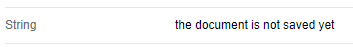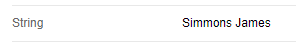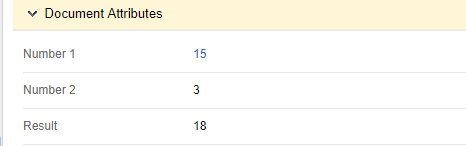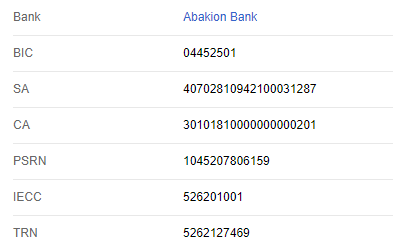# Calculatable fields when changing document attributes

Important!Attribute values are calculated when creating, saving and changing (after clicking Save) a document.

## Example 1

This example shows how to save the value of the Contractor Name variable to a string. You will need to create a String attribute of the String type, and add it to the Value Calculation Script field of the attribute. Also, add a Contractor attribute of the Contractor type. The value will be written to the String attribute.Fig. 1. Document attributes

Script:

``String = (Contractor != null) ? Contractor.Name : "the contractor has no name";``

If the contractor field is empty, the message "the contractor has no name" will be displayed (fig. 2), otherwise (fig. 3).Fig. 2. The value if the contractor field is emptyFig 3. The value after selecting a contractor and saving the document.

## Example 2

In this example, the document author name is written to an attribute. You will need to create a String attribute of the String type, and add it to the Value Calculation Script field of the attribute. The Author (CreationAuthor) variable is created automatically in each document type.

Script:

``String = (CreationAuthor != null) ? CreationAuthor.ToString() : "the document is not saved yet";``

Before saving the document, the Author field is empty, therefore the String attribute will display the message "the document is not saved yet (fig. 4). After saving the document, the Author attribute will be filled in and the username will be inserted in the String field (fig. 5.).Fig. 4. String value before saving the document.Fig. 5. String value after saving the document.

## Example 3

In this example, the sum of two numbers is calculated. You will need to add the following attributes: Number 1, Number 2, Result of the Integer type, add the script to the Value Calculation Script field. The document attributes are shown in fig. 6.

For the script to work correctly, make the Number1 and Number2 variables required. After filling in the variables, their sum will be calculated. In a similar way, you can implement any calculation logic.Fig. 6. Document attributes.

Script:

``Result = ((Number1 != null) && (Number2 != null)) ? Number1+Number2: 0;``

Fig. 7 and 8 show how the script works.Fig. 7. Value before saving the document.Fig 8. Value after saving the document.

## Example 4

In this example, a string displays the full name of the selected user from a context variable of the User type. The script is added to the Full Name variable of the String type, in the Value Calculation Script field.Fig. 9. Document attributes.

Script:

``FullName = (User != null) ? User.FullName: "user not selected";``

If the User attribute is filled in, then the Full Name field will display the full name of the selected user (fig. 11), otherwise, the message "user not selected" will be displayed (fig. 10).Fig. 10. Value before saving the document.Fig. 11. Value after saving the document.

## Example 5

In this example, when creating a document of the Contract type, the company details are inserted automatically. The script is added to the String-type variables; attributes:  Bank (Bank), BIC (BIC), SA (SA), CA (CA), PSRN (PSRN), IECC (IECC), TRN (TRN), the scripts are added to the Value Calculation Script fields. The data are specified in the attributes manually. This example shows the value calculation when creating, not saving the document.Fig. 12. Document attributes

Script:

``````Bank = "Abakion Bank";
BIC = "044525201";
SA = "40702810942100031287";
CA = "30101810000000000201";
PSRN = "1045207806159";
IECC = "526201001";
TRN = "5262127469";``````

Result:Fig. 13. Value when creating the document.

## Example 6

A script for calling a complex function in a calculatable field:

``````new Func<string>(() =>{
string res = "";